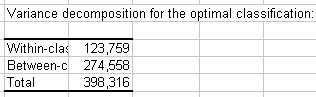# k-means clustering in Excel tutorial

2017-12-19

This tutorial will help you set up and interpretk-means Clustering in Excel using the XLSTAT software.
Not sure if this is the right clustering tool you need? Check out this guide.

## Dataset for k-means clustering

An Excel sheet with both the data and the results can be downloaded by clicking on the button below:

The data are from the US Census Bureau and describe the changes in the population of 51 states between 2000 and 2001. The initial dataset has been transformed to rates per 1000 inhabitants, with the data for 2001 serving as the focus for the analysis. Our aim is to create homogeneous clusters of states based on the demographic data we have available.

Note: if you try to re-run the same analysis as described below on the same data, as the k-means method starts from randomly selected clusters, you will most probably obtain different results from those listed hereunder, unless you fix the seed of the random numbers to the same value as the one used here (910837696). To fix the seed, go to the XLSTAT Options, Advanced tab, then check the "fix the seed" option.

## Setting up a k-means clustering

Once XLSTAT is activated, go to XLSTAT / Analyzing data / k-means clustering.Once you've clicked the button, the k-means clustering dialog box appears. Then select the data on the Excel sheet with the mouse.

Note: There are several ways of selecting data with XLSTAT - for further information, please check the tutorial on selecting data.

In this example, the data start from the first row, so it is quicker and easier to use the "column selection" mode. This explains why the letters corresponding to the columns are displayed in the selection boxes.

The "Total population" variable was not selected, as we are interested mainly in the demographic dynamics. The last column was not selected as it is fully correlated with the column preceding it.

The observations labels were selected as they are available. We set the number of groups to create to 4. The selected criterion is Determinant(W) as it allows to remove the scale effects of the variables.In the options tab we increased the number of repetitions to 50 in order to increase the quality and the stability of the results.## Interpreting a k-means clustering

After the basic descriptive statistics of the selected variables, the first result displayed is the optimization summary and the Initial within-class variances and Final within-class variances.

We notice that the optimal solution for the selected criterion does not mimimize the within-class variance (123.76, when the minimum is less than 113.27). This is because the criterion is not the within variance itself, but the determinant of W, where W is the within-class variance matrix.

The variance decomposition table for the best solution among the repetitions is displayed. (Note: Total variance = Between-classes variance + Within-class variance.)After a series of tables that include the class centroids, the distance between the class centroids, the central objects (here, the state that is the closest to the class centroid), a table shows the states that have been classified into each cluster.Then a table with the group ID for each state is displayed. A sample is shown below. The cluster IDs can be merged (or joined) with the initial table for further analyses (discriminant analysis for example.)In the tutorial on Agglomerative Hierarchical Clustering (AHC), we see that the States would better be clustered into three groups.

This video shows you how to group samples with the k-means clustering.

#### Contact our technical support team: support@xlstat.com

https://cdn.desk.com/
false
desk